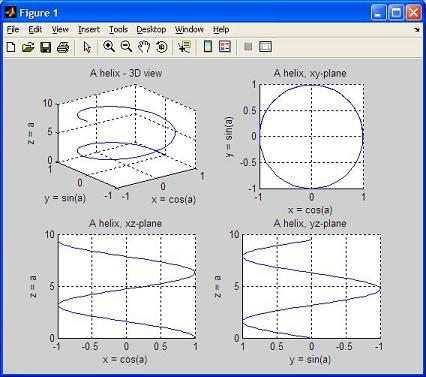# 3D Plot – Part 1

From '3D Plot Part 1' to 3D Main

Matlab provides many powerful instructions for the visualization of 3D data.

The instructions provided include tools to plot wire-frame objects, 3D plots, curves, surfaces...

... and can automatically generate contours, display volumetric data, interpolate shading colors and even display non-Matlab made images. Here are some commonly used functions (there are many more):
• plot3
• stem3
• pie3
• comet3
• contour3
• mesh
• meshc
• surf
• surfc
• sphere
• ellipsoid
• cylinder

Among these instructions, plot3 and comet3 are the 3D matches of plot and comet commands mentioned in the 2D plot section.

The general syntax for the plot3 command is
plot3(x, y, z, 'style')

This command draws a 3D curve with the specified line style. The argument list can be repeated to make overlay plots, just the same way as with the plot command in 2D.

We have to make some necessary comments before any example can be introduced.

Plots in 3D may be annotated with instructions already mentioned for 2D plots: xlabel, ylabel, title, legend, grid, etc., plus the addition of zlabel. The grid command in 3D makes the appearance of the plots better, especially for curves in space.

View
The viewing angle of the observer is specified by the command view(azimuth, elevation), where
• azimuth (in degrees): specifies the horizontal rotation from the y-axis, measured positive counterclockwise (default value is -37.5 degrees).
• elevation (in degrees): specifies the vertical angle measured positive above the xy-plane (default value is 30 degrees).By specifying appropriate values of azimuth and elevation, one can plot projections of 3D objects on different 2D planes. For example, the command 'view(90,0)' places the viewer toward the positive x-axis, looking straigth on the yz-plane, and thus produces a 2D projection of the object on the yz-plane. 'view(0, 90)' shows the figure on a 2D xy-plane.

The following script generates data, plots the curves and obtains different views.

Example:

% clears variables, command window and closes all previous figures
clear; clc; close all

% generates an angle vector with 101 values
a = 0: 3*pi/100 : 3*pi;

% calculates x, y, and z
x = cos(a);
y = sin(a);
z = a;

% divides the figure window into 4 subwindows (2x2)
% plots on the 1st. one
subplot(2,2,1)
plot3(x,y,z)
grid on
title('A helix - 3D view')
xlabel('x = cos(a)')
ylabel('y = sin(a)')
zlabel('z = a')

% plots on the 2nd. subwindow
subplot(2,2,2)
plot3(x,y,z)
axis('square')
% rotates the figure to show only the xy-plane
view(0,90)
grid on
title('A helix, xy-plane')
xlabel('x = cos(a)')
ylabel('y = sin(a)')
zlabel('z = a')

% plots on the 3rd. subwindow
subplot(2,2,3)
plot3(x,y,z)
% rotates the figure to show only the xz-plane
view(0,0)
grid on
title('A helix, xz-plane')
xlabel('x = cos(a)')
ylabel('y = sin(a)')
zlabel('z = a')

% plots on the 4th. subwindow
subplot(2,2,4)
plot3(x,y,z)
% rotates the figure to show only the yz-plane
view(-90,0)
grid on
title('A helix, yz-plane')
xlabel('x = cos(a)')
ylabel('y = sin(a)')
zlabel('z = a')

And the result is:Matlab-Examples home

From '3D Plot Part 1' to 3D Main Menu

 Part 2 - meshgrid, plot3, meshc Part 3 - plot3, sphere, mesh Part 4 - surfaces, meshgrid, contour3 Top